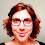## Friday, February 15, 2013

### Function to get the sister(s) of a node or tip

I just posted a new utility function, getSisters, that takes as input a tree and a node or tip number or label, and returns the sister node or tip numbers or labels. It has two modes: mode="number", which returns node or tip numbers as an integer or vector; and mode="label" which returns a list with up to two components - one component for node labels (if available) or numbers, and the other component with tip labels.

The code for the function is here, but it also in the most recent build of phytools: phytools 0.2-18.

Here's a quick demo:
> require(phytools)
> tree<-rtree(n=12)
> plotTree(tree,node.numbers=TRUE)> getSisters(tree,19)
 23
> getSisters(tree,18,mode="label")
\$tips
 "t10"

You get the idea. We can also collapse some branches so that some tips or nodes have multiple sister tips or nodes:
> tree\$edge.length[which(tree\$edge[,2]==18)]<-0
> tree\$edge.length[which(tree\$edge[,2]==23)]<-0
> tree\$edge.length[which(tree\$edge[,2]==20)]<-0
> tree\$edge.length[which(tree\$edge[,2]==21)]<-0
> tree<-di2multi(tree)
> plotTree(tree,node.numbers=T)> getSisters(tree,18,mode="label")
\$tips
 "t8" "t2" "t10"

> getSisters(tree,"t4",mode="label")
\$nodes
 19

\$tips
 "t3" "t11"

That's it.

1.A useful little utility function, thanks Liam!

Thought I'd just post a little code I use with this utility function to find all of the sister species pairs in a tree (Only works for fully resolved, but can be easily modified for polytomies)

# find only sister-species pairs
sisters <- matrix(NA, ncol=2, nrow=length(tree\$tip.label)) # empty matrix
sisters[,1] <- tree\$tip.label # populate first column with tip.labels
for(i in 1:length(tree\$tip.label)){
tmp <- phytools::getSisters(tree, tree\$tip.label[i], mode="label")
if(!is.null(tmp\$tips)){sisters[i,2] <- tmp\$tips}
}
sisters <- sisters[-which(is.na(sisters[,2])),] # prune away tips that do not have a labelled tip as sister

1.Hi Emma. sisters is redundant with functions in phangorn, which are probably faster.

You might get a table with all pairs of sister species in a bifurcating tree using phangorn & apply family functions as follows:

dd<-lapply(1:tree\$Nnode+Ntip(tree),function(n,t)
Descendants(t,n)[],t=tree)
nodes<-c(1:tree\$Nnode+Ntip(tree))[which(sapply(dd,length)==2)]
sisters<-t(sapply(nodes,function(n,t)
t\$tip.label[Descendants(t,n)[]],t=tree))

- Liam

2.Hi Liam,

This is really useful. Is it possible to get all the sister species from a multiphylo object?

3.Note: due to the very large amount of spam, all comments are now automatically submitted for moderation.